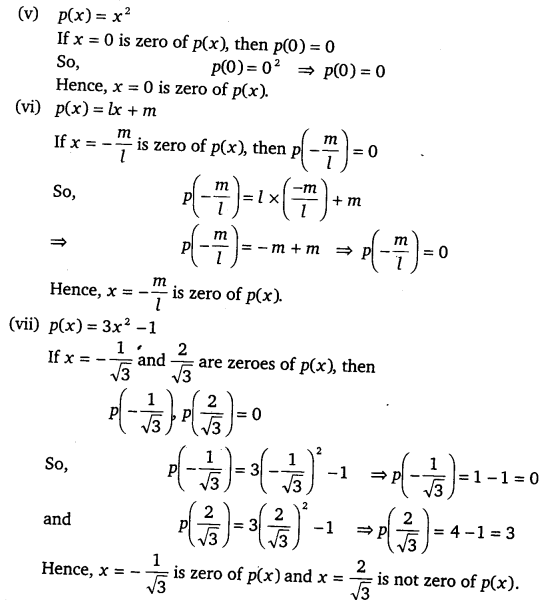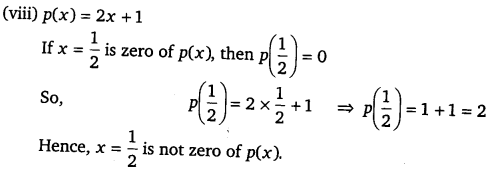# CLASS 9 MATH NCERT SOLUTION FOR CHAPTER – 2 POLYNOMIALS EX – 2.2

## Polynomials

Question 1.
Find the value of the polynomial 5x – 4x2 + 3 at
(i) x=0
(ii) x=-1
(iii) x=2

Solution:

Question 2.
Find p(0), p(1) and p(2) for each of the following polynomials
(i) p(y)=y2-y+1
(ii) p(t) =2+t+2t2-t3
(iii) p(x) =x3
(iv) p (x)=(x-1)(x+1)

Solution:

(i) We have,p(y) = y2-y+1
∴ p(0)= (0)2-0+1 = 0-0+1 =1,
p(1)=(1)2 -1+1 = 1-1+1 = 1,
and  p(2) = (2)2 – 2 +1 = 4 – 2 +1 = 3

(ii) We have,    p(t) = 2 +1 + 2t2 -t3
∴  p(0) = 2 + 0 + 2(0)2 – (0)3
= 2 + 0+ 0-0 = 2,
p(1) = 2 +1 + 2(1)2 – (l)3
= 2+l+2-l = 5-1 = 4
p(2) = 2 + 2 + 2(2)2 – (2)3
= 2 + 2 + 8-8 = 4

(iii) We have, p(x) = x3
p(o) = (0 )3 = 0,
p(1)=(1)3 =1,
p(2) = (2)3 = 8

(iv) p(x) = (x-1) (x +1)
p(0) = (0 -1)(0 +1) = (-1)(1) = -1
p(1) = (2 -1) (1 +1) = (0)(2) = 0
p(2) = (2-1) (2 +1) = (1)(3) = 3

Question 3.
Verify whether the following are zeroes of the polynomial, indicated against them.
(i) p(x) =3x+1,x=-$\cfrac { 1 }{ 3 }$
(ii) p(x)=5x-π,x=$\cfrac { 4 }{ 5 }$
(iii) p(x) =x2 -1,x=1,-1
(iv) p(x)=(x+1)(x-2),x = -1,2
(v) p(x) =x,x=0
(vi) p(x)=lx+m,x = –$\cfrac { m }{ l }$
(vii) p(x) =3x-1,x= –$\cfrac { 1 }{ \sqrt { 3 } }$ ,$\cfrac { 2 }{ \sqrt { 3 } }$
(viii) p(x) = 2x+1,x$\cfrac { 1 }{ 2 }$

Solution:Question 4.
Find the zero of the polynomial in each of the following cases :
(i) p(x) = x + 5
(ii) p(x) = x – 5
(iii) p(x) = 2x + 5
(vi) p(x) = 3x-2
(v) p(x) = 3x
(vi) p(x) = ax, a≠0
(vii) p(x)  = cx + d, c≠0, c, d are real numbers.

Solution:

(i) We have, p(x) = x + 5
Now,   p(x) = 0      => x + 5 = 0 => x = -5
∴ 5 is a zero of the polynomial p(x).

#### CLASS 9 MATH NCERT SOLUTION FOR CHAPTER – 2 POLYNOMIALS EX – 2.3

Lorem ipsum dolor sit amet, consectetur adipiscing elit. Phasellus cursus rutrum est nec suscipit. Ut et ultrices nisi. Vivamus id nisl ligula. Nulla sed iaculis ipsum.

Company Name